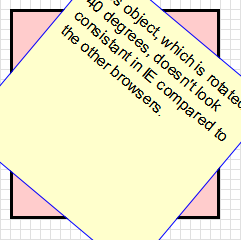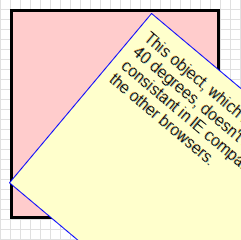Below are some notes on the differences between CSS3 Transforms and IE's Matrix Visual Filter. Information about each of the CSS3 Transform functions are available on the links at the side. Go back to the Transforms Translator.

## Supported Transform Functions

This tool written by Zoltan Hawryluk and Zoe Mickley Gillenwater.

Powered by CSS Sandpaper, with the help of code written by Weston Ruter, Dean Edwards and Andrew Johnson.

This tool converts CSS3 Transform properties (which almost all modern browsers use) to the equivalent CSS using Microsoft's proprietary Visual Filters technology.

## Differences Between the IE Matrix filter and CSS3 Transforms

1. CSS3 transforms, by default, do transformations with respect to the center of the object, while the Matrix filter tries to fit the transformed object in the smallest amount of space possible, as seen by the illustration below:
Rotate using CSS Transform Rotate using IE Matrix FilterIn order to work around this difference, the IE Transform Translator uses the width and height values to move the transformed object the same position that a CSS3 Transformation would, using the margin-left and margin-top properties.
2. If you want to ensure consistent positioning of the transformed element, use IE with this tool. The other browsers make a very good mathematical guess on the values of  margin-left  and  margin-top, while IE calculates them more accurately. However, even when using IE, you may, from time to time, find you will be off by a few pixels. This may be due to other CSS rules in your style sheet.
3. If you do use a Matrix filter on an HTML element, and then use another Matrix filter on a child node of that element, you may get unexpected results (usually, the transformed element is cropped unexpectedly).
4. Scaling in IE is not as good as scaling in browsers that do native CSS3 transforms. It seems that this is because IE's Matrix filter transforms a raster version of the original element, while the other browsers seem to transform a vector version (For those who are not sure what the difference is, the article An explanation of Raster vs Vector will explain).
5. In IE, users can select the text with a mouse inside a transformed element more easily if the element is not scaled or flipped upside down. Skewing, or performing multiple transforms on an element gives mixed results.
6. The order of the parameters of IE's Matrix filter must be exactly as they are in the tool, especially the SizingMethod parameter. Doing otherwise may prevent other Matrix filters in the same style sheet to work correctly.
7. If you want to set transforms using JavaScript (i.e. doing animations), it is better to use a library like cssSandpaper, because of the many quirks that happen when scripting Visual Filters (it is a real pain).
8. It is best to use IE's Matrix filter with solid backgrounds (i.e. a solid color or a image that doesn't have a transparency or alpha channel), otherwise the result will be a little pixelated. Update: If you are transforming an object with a background that isn't a solid color, you need to add a Chroma filter before you apply the Matrix filter.
9. The -ms-filter property must be before the corresponding filter rule. The value in quotes must also be contained in one line.
10. If combining IE's Matrix filter with other filters in the same CSS selector (e.g. the  gradient  filter), it is important to put all the filters into one CSS rule:
#transformedObject {

/* IE8 - must be on one line. */
-ms-filter: "progid:DXImageTransform.Microsoft.gradient(startColorStr='#99B4B490',EndColorStr='#99B4B490') progid:DXImageTransform.Microsoft.Matrix(M11=0.9914448613738104, M12=-0.13052619222005157, M21=0.13052619222005157, M22=0.9914448613738104, SizingMethod='auto expand')";

/* IE6,IE7 - can span multiple lines */
startColorStr='#99B4B490',EndColorStr='#99B4B490')
progid:DXImageTransform.Microsoft.Matrix(
M11=0.9914448613738104, M12=-0.13052619222005157,
M21=0.13052619222005157, M22=0.9914448613738104,
sizingMethod='auto expand');

}


## Step 2: Copy and paste the cross-browser CSS!

For CSS3 Savvy Browsers For IE





To ensure you're transforms will look optimal in IE, please take a look at our user notes.

## rotate( angle)

Rotates HTML elements. The  angle  can be in degrees (e.g. rotate(30deg)) or radians (e.g. rotate(1.3rad))

 rotate(45deg) 

This is the object before transformation.

This is the object after transformation.

## scale( sx, sy)

Scales HTML elements. The parameters  sx  and  sy  are numbers, where  1  represents the original size,  2  represents twice the size,  0.5  represents half the size, etc. Note that if  sy  isn’t specified, it is assumed to be equal to  sx  . Related functions are  scaleX(sx)  (scales horizontally only) and  scaleY(sy)  (scales vertically only).

Original  scaleX(1.3)   scaleY(1.3)   scale(0.5, 0.5)   scale(-1, 1)   scale(1, -1)Image Source: Wikimedia Commons.

## skew( x-angle, y-angle)

Skews the object around the x and y axes by the specified angles in degrees or radians. If  ay  isn’t provided, it is assumed to be  0deg 

 skewX(25deg)   skewY(35deg)   skew(25deg, 35deg) 

This is the object before transformation.

This is the object after transformation.

This is the object before transformation.

This is the object after transformation.

This is the object before transformation.

This is the object after transformation.

## translate( tx, ty)

Moves the object tx horizontally and ty vertically.

 translateX(40px)   translateY(30px)   translate(40px, 30px) 

This is the object before transformation.

This is the object after transformation.

This is the object before transformation.

This is the object after transformation.

This is the object before transformation.

This is the object after transformation.

## matrix( a, c, b, d, tx, ty)

This is the hardest of all the transform functions to understand unless you are mathematically gifted. However, for those who are stubborn, geeky, or both, a brief explanation follows. If you don't undertand everything below, don't fret — you'll probably never use this function.

This function is almost the direct equivalent to Microsoft's Matrix filter. These first four values,  a  ,  c  ,  b  and  d  , correspond to the first four values of Microsoft Matrix filter,  M11  ,  M12  ,  M21  and  M22  . In other words, this statement

transform: matrix(1, 2, 3, 4, 0, 0);

is the same as this statement:
filter: progid:DXImageTransform.Microsoft.Matrix(
M11=1,
M12=3,
M21=2,
M22=4,
SizingMethod='auto expand');

Note that  c  and  b  are out of order. This is due to a difference in Microsoft's convention compared to the W3C's.

The last two numbers,  tx  and  ty  , are the translation factors for the object. In other words, this statement:

transform: matrix(1, 0, 0, 1, 22px, 33px);


is the same as this statement

transform: translate(22px, 33px);


and this mathematical notation:

Note: at the time of this writing, Firefox (version 3.6) requires units for  tx  and  ty  (such as  px  or  em  ) which Safari 4, Opera 10.10 and Chrome 5.0 require units not be supplied. At this time, this tool does not take this into account.

As mentioned earlier, if you aren’t familiar with linear algebra and matrix arithmetic, this function will be hard to understand. That's okay, because most designers will never need this function. For further information, you may want to read Wikipedia’s Transformation Matrix article, although if you are mathematically challenged, you may run away from your computer screaming.